##全国校区# python分析6625条视频，揭秘“打工人”凭啥刷爆全网！2020-11-02CDA数据分析师 出品

【导读】

Show me data，用数据说话！今天我们聊一聊 python分析“打工人”

“累吗？累就对了，舒服是留给有钱人的。早安，打工人！冷吗？冷就对了，温暖是留给开小轿车的人。早安，打工人！”01、数据读入

```# 导入包
import numpy as np
import pandas as pd
import matplotlib.pyplot as plt
```
```# 读入数据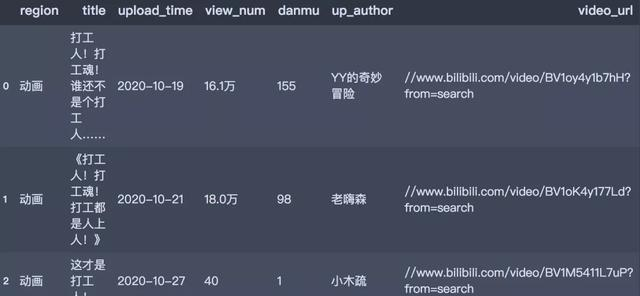```print(df.shape)
(6625, 7)```

02数据预处理

• 去除重复值
• view_num和danmu：单位转换
• 筛选数据

```def transform_unit(x_col):
"""
功能：转换数值型变量的单位
"""
# 提取数值
s_num = df[x_col].str.extract('(d+.*d*)').astype('float')
# 提取单位
s_unit = df[x_col].str.extract('([u4e00-u9fa5]+)')
s_unit = s_unit.replace('万', 10000).replace(np.nan, 1)
s_multiply = s_num * s_unit
return s_multiply```
```# 去重
df = df.drop_duplicates()

# 删除列
df.drop('video_url', axis=1, inplace=True)

# 转换单位
df['view_num'] = transform_unit(x_col='view_num')
df['danmu'] = transform_unit(x_col='danmu')

# 筛选时间
df = df[(df['upload_time'] >= '2020-09-01') & (df['title'].astype('str').str.contains('打工人'))]03、数据可视化分析

```import jieba
from pyecharts.charts import Bar, Line, Pie, Map, Scatter, Page
from pyecharts import options as opts
from pyecharts.globals import SymbolType, WarningType
WarningType.ShowWarning = False```

01 打工人视频发布热度走势图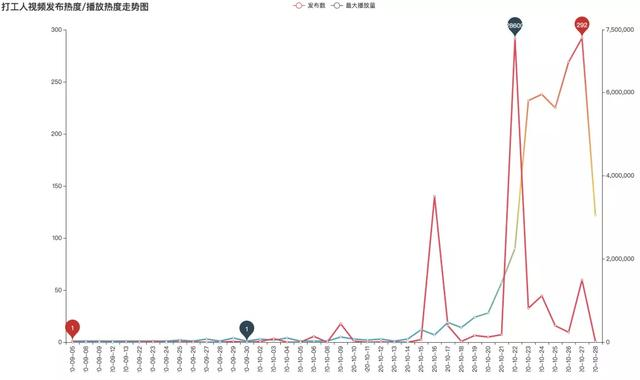10月16日，up主“老摸鱼艺术家”的《加油！打工人！》播放量突破350万。几天后，10月22日，up主“三Lu有毒”的视频《早安，打工人！》更是加上了各种打工人优秀语录，同时配上魔性的画面和声音，直接在B站爆火，目前该视频播放量已突破913万。

```time_num = df.upload_time.value_counts().sort_index()
time_num[:5]
2020-09-05    1
2020-09-08    1
2020-09-09    1
2020-09-12    1
2020-09-13    1
```# 条形图
line1 = Line(init_opts=opts.InitOpts(width='1350px', height='750px'))
markpoint_opts=opts.MarkPointOpts(data=[opts.MarkPointItem(type_='min'),
opts.MarkPointItem(type_='max')])
)
line1.set_global_opts(title_opts=opts.TitleOpts(title='打工人视频发布热度走势图', pos_left='40%'),
xaxis_opts=opts.AxisOpts(axislabel_opts=opts.LabelOpts(rotate='90')),
visualmap_opts=opts.VisualMapOpts(max_=int(time_num.max()), is_show=False),
)
line1.set_series_opts(linestyle_opts=opts.LineStyleOpts(width=3),
label_opts=opts.LabelOpts(is_show=False)
)
line1.render() ```

02 不同分区的视频发布数量03 不同分区的视频发布播放量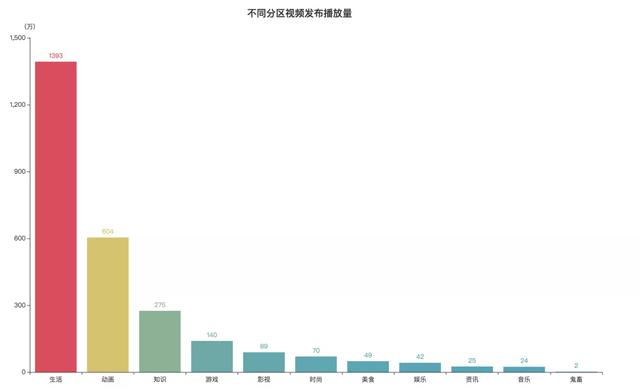04 最高播放的Top10视频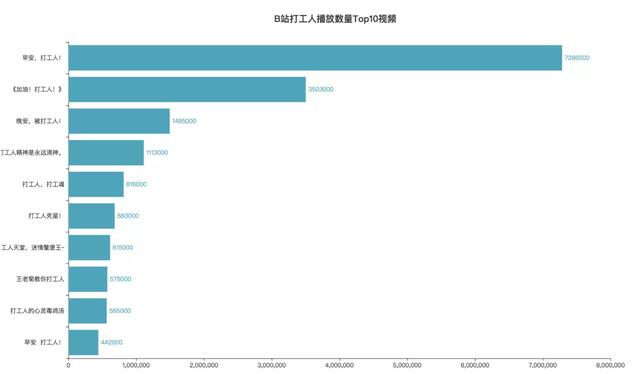05 早安，打工人！弹幕词云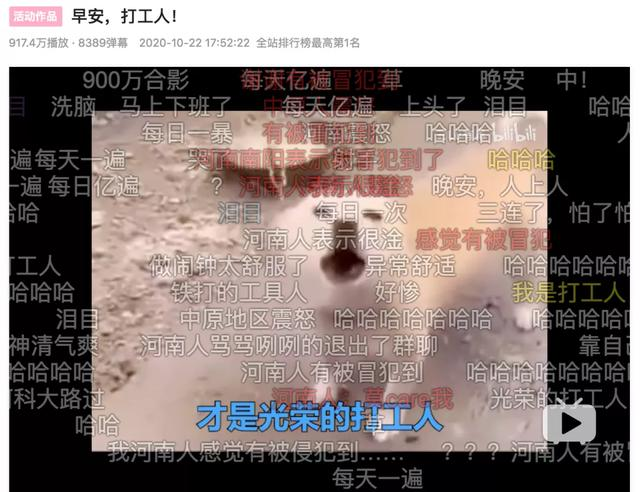06 《加油，打工人！》弹幕词云“加油”、“打工人”、“真实”等都是妥妥的高频词。配上最近常被up用来二次创造的动画片《校园小子》，有“文艺复兴”那味儿了。

07 打工人标题词云图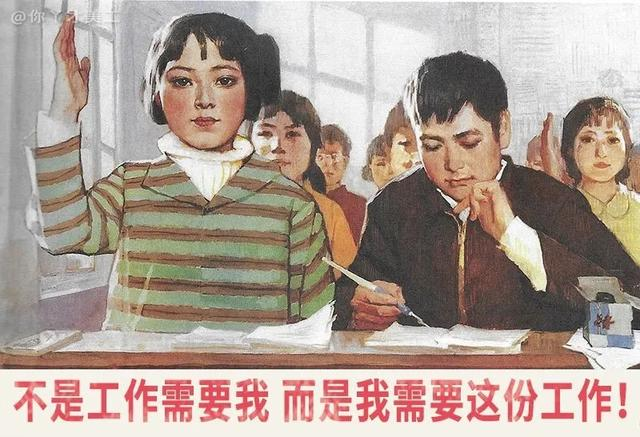```
——热门课程推荐：

想从事业务型数据分析师，您可以点击>>>“数据分析师”了解课程详情；

想从事大数据分析师，您可以点击>>>“大数据就业”了解课程详情；

想成为人工智能工程师，您可以点击>>>“人工智能就业”了解课程详情；

想了解Python数据分析，您可以点击>>>“Python数据分析师”了解课程详情；

想咨询互联网运营，你可以点击>>>“互联网运营就业班”了解课程详情；

想了解更多优质课程，请点击>>>

```

OK